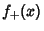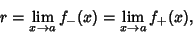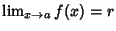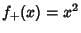## Squeezing TheoremLet there be two functionsandsuch thatis squeezed'' between the two,Ifthen. In the above diagram the functionsandsqueeze''at 0, so. The squeezing theorem is also called the Sandwich Theorem.